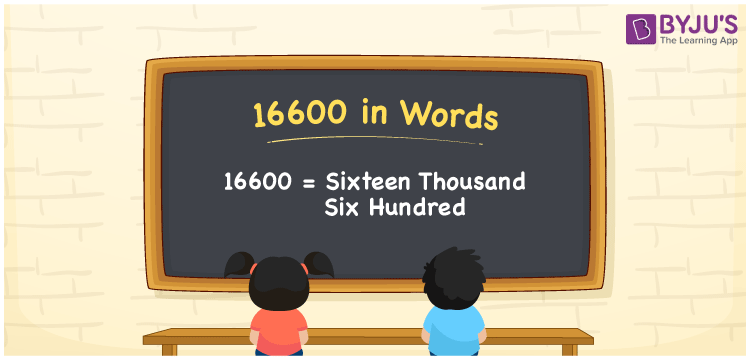# 16600 in Words

Sixteen Thousand Six Hundred is the numerical name for the number 16600. For example, if you donated Rs. 16600 to an old age home, then you can write, “I donated Rupees Sixteen Thousand Six Hundred to an old age home”. We know 16600 is a cardinal number since it shows an exact quantity. Place value chart plays an important role in determining the numerical name easily. This article will provide information about how to convert the number 16600 into words.

 16600 in Words Sixteen Thousand Six Hundred Sixteen Thousand Six Hundred in numerical form 16600

## 16600 in English Words

English is the most widely used language in education. Therefore, we generally write the numbers in words using the letters of the English alphabet. Hence, we can express the number 16600 in English as Sixteen Thousand Six Hundred.## How to Write 16600 in Words?

With the help of the place value chart, it is easy to write the numerical name by identifying the position of each digit in the given number. The following table shows the place value chart for the number 16600.

 Ten-Thousands Thousands Hundreds Tens Ones 1 6 6 0 0

Therefore, we can write the expanded form as:

1 x Ten Thousand + 6 x Thousand + 6 x Hundred + 0 x Ten + 0 x One

= 1 x 10000 + 6 x 1000 + 6 x 100 + 0 x 10 + 0 x 1

= 10000 + 6000 + 600 + 0 + 0

= 10000 + 6000 + 600 + 0

= 10000 + 6000 + 600

= 16600

= Sixteen Thousand Six Hundred

Hence, 16600 in words is written as Sixteen Thousand Six Hundred

Interesting way of writing 16600 in words

1 = One

16 = Sixteen

166 = One Hundred and Sixty-Six

1660 = One Thousand Six Hundred Sixty

16600 = Sixteen Thousand Six Hundred

Thus, the word form of the number 16600 is Sixteen Thousand Six Hundred

16600 is a natural number that is the successor of 16599 and the predecessor of 16601.

• 16600 in words – Sixteen Thousand Six Hundred
• Is 16600 an odd number? – No
• Is 16600 an even number? – Yes
• Is 16600 a perfect square number? – No
• Is 16600 a perfect cube number? – No
• Is 16600 a prime number? – No
• Is 16600 a composite number? – Yes

## Frequently Asked Questions on 16600 in Words

Q1

### How do you write 16600 in words?

We write 16600 in words as Sixteen Thousand Six Hundred.
Q2

### Simplify 15000 + 1600, and express in words.

Simplifying 15000 + 1600, we get 16600. Therefore, the number 16600 in words is Sixteen Thousand Six Hundred.
Q3

### 16600 is an even number. True or False.

True, 16600 is an even number.